Total Pressure

 |   Tiled Menu   |   Tables of Content   |  Banner Video   |  Tohline Home Page   |

In our overview of equations of state, we identified analytic expressions for the pressure of an ideal gas,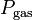$~P_\mathrm{gas}$, electron degeneracy pressure,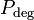$~P_\mathrm{deg}$, and radiation pressure,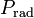$~P_\mathrm{rad}$. Rather than considering these relations one at a time, in general we should consider the contributions to the pressure that are made by all three simultaneously. That is, we should examine the total pressure,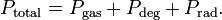$~P_\mathrm{total} = P_\mathrm{gas} + P_\mathrm{deg} + P_\mathrm{rad} .$

In order to assess which of these three contributions will dominate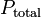$~P_\mathrm{total}$ in different density and temperature regimes, it is instructive to normalize$~P_\mathrm{total}$ to the characteristic Fermi pressure,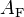$~A_\mathrm{F}$, as defined in the accompanying Variables Appendix. As derived below, this normalized total pressure can be written as,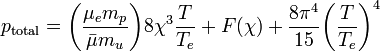$~p_\mathrm{total} = \biggl(\frac{\mu_e m_p}{\bar{\mu} m_u} \biggr) 8 \chi^3 \frac{T}{T_e} + F(\chi) + \frac{8\pi^4}{15} \biggl( \frac{T}{T_e} \biggr)^4$

Derivation

We begin by defining the normalized total gas pressure as follows: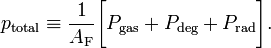$p_\mathrm{total} \equiv \frac{1}{A_\mathrm{F}} \biggl[ P_\mathrm{gas} + P_\mathrm{deg} + P_\mathrm{rad} \biggr] .$

To derive the expression for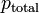$~p_\mathrm{total}$ shown in the opening paragraph above, we begin by normalizing each component pressure independently.

Normalized Degenerate Electron Pressure

This normalization is trivial. Given the original expression for the pressure due to a degenerate electron gas (or a zero-temperature Fermi gas),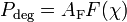$~P_\mathrm{deg} = A_\mathrm{F} F(\chi)$ where: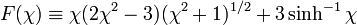$F(\chi) \equiv \chi(2\chi^2 - 3)(\chi^2 + 1)^{1/2} + 3\sinh^{-1}\chi$ and: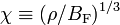$\chi \equiv (\rho/B_\mathrm{F})^{1/3}$

we see that,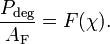$\frac{P_\mathrm{deg}}{A_\mathrm{F}} = F(\chi) .$

Normalized Ideal-Gas Pressure

Given the original expression for the pressure of an ideal gas,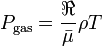$~P_\mathrm{gas} = \frac{\Re}{\bar{\mu}} \rho T$

along with the definitions of the physical constants,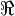$~\Re$,$~A_\mathrm{F}$, and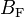$~B_\mathrm{F}$ provided in the accompanying Variables Appendix, we can write,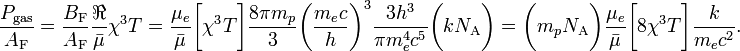$\frac{P_\mathrm{gas}}{A_\mathrm{F}} = \frac{B_\mathrm{F}}{A_\mathrm{F}} \frac{\Re}{\bar{\mu}} \chi^3 T = \frac{\mu_e}{\bar{\mu}} \biggl[ \chi^3 T \biggr] \frac{8\pi m_p}{3} \biggl( \frac{m_e c}{h} \biggr)^3 \frac{3h^3}{\pi m_e^4 c^5} \biggl(k N_\mathrm{A} \biggr) = \biggl(m_p N_\mathrm{A} \biggr)\frac{\mu_e}{\bar{\mu}} \biggl[8 \chi^3 T \biggr] \frac{k}{ m_e c^2} .$

Therefore, letting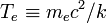$T_e \equiv m_e c^2/k$ represent the temperature associated with the rest-mass energy of the electron, the normalized ideal gas pressure is,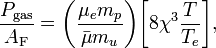$\frac{P_\mathrm{gas}}{A_\mathrm{F}} = \biggl(\frac{\mu_e m_p}{\bar{\mu} m_u} \biggr) \biggl[8 \chi^3 \frac{T}{T_e} \biggr] ,$

where, by definition, the atomic mass unit is,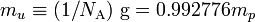$m_u \equiv (1/N_\mathrm{A})~\mathrm{g} = 0.992776 m_p$, that is,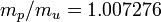$~m_p/m_u = 1.007276$.

Given the original expression for the radiation pressure,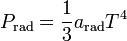$~P_\mathrm{rad} = \frac{1}{3} a_\mathrm{rad} T^4$

along with the definitions of the physical constants,$~A_\mathrm{F}$, and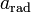$~a_\mathrm{rad}$ provided in the accompanying Variables Appendix, we can write,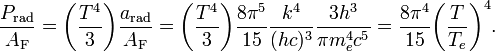$\frac{P_\mathrm{rad}}{A_\mathrm{F}} = \biggl( \frac{T^4}{3} \biggr) \frac{a_\mathrm{rad}}{A_\mathrm{F}} = \biggl( \frac{T^4}{3} \biggr) \frac{8\pi^5}{15}\frac{k^4}{(hc)^3} \frac{3h^3}{\pi m_e^4 c^5} = \frac{8\pi^4}{15} \biggl( \frac{T}{T_e} \biggr)^4 .$

Discussion

For simplicity of presentation, in what follows we will use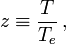$z \equiv \frac{T}{T_e} \, ,$

to represent a normalized temperature, in addition to using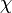$~\chi$ to represent (the cube root of) the normalized mass density, and$~p_\mathrm{total}$ to represent the normalized total pressure.

Relationship Between State Variables

If the two normalized state variables,$~\chi$ and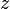$~z$, are known, then the third normalized state variable,$~p_\mathrm{total}$, can be obtained directly from the above key expression for the total pressure, that is,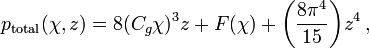$p_\mathrm{total}(\chi, z) = 8(C_g \chi)^3 z + F(\chi) + \biggl(\frac{8\pi^4}{15}\biggr) z^4 \, ,$

where,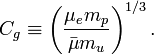$C_g \equiv \biggl(\frac{\mu_e m_p}{\bar\mu m_u}\biggr)^{1/3} \, .$

If it is the two normalized state variables,$~\chi$ and$~p_\mathrm{total}$, that are known, the third normalized state variable — namely, the normalized temperature,$~z$ — also can be obtained analytically. But the governing expression is not as simple because it results from an inversion of the total pressure equation and, hence, the solution of a quartic equation. As is detailed in the accompanying discussion, the desired solution is,$z(\chi, p_\mathrm{total}) = \theta_\chi \phi^{-1/3}\biggl[ (\phi - 1)^{1/2} - 1 \biggr] ,$

where,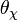$~\theta_\chi$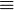$~\equiv$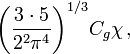$~\biggl( \frac{3\cdot 5}{2^2 \pi^4} \biggr)^{1/3} C_g\chi \, ,$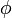$~\phi$$~\equiv$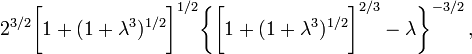$~ 2^{3/2} \biggl[ 1 + (1 + \lambda^3)^{1/2} \biggr]^{1/2} \biggl\{ \biggl[ 1 + (1 + \lambda^3)^{1/2} \biggr]^{2/3} - \lambda \biggr\}^{-3/2}\, ,$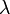$~\lambda$$~\equiv$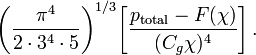$~ \biggl(\frac{\pi^4}{2\cdot 3^4\cdot 5} \biggr)^{1/3} \biggl[\frac{p_\mathrm{total}-F(\chi)}{(C_g \chi)^{4}}\biggr] \, .$

It also would be desirable to have an analytic expression for the function,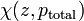$~\chi(z, p_\mathrm{total})$, in order to be able to immediately determine the normalized density from any specified values of the normalized temperature and normalized pressure. However, it does not appear that the above key expression for the total pressure can be inverted to provide such a closed-form expression.

Dominant Contributions

Let's examine which pressure contributions will dominate in various temperature-density regimes. Note, first, that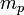$~m_p$/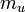$~m_u$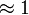$~\approx 1$ and, for fully ionized gases, the ratio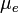$~\mu_e$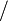$~/$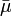$~\bar{\mu}$ is of order unity — more precisely, the ratio of these two molecular weights falls within the narrow range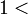$~1 <$$~\mu_e$$~/$$~\bar{\mu}$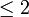$\le 2$. Hence, we can assume that the numerical coefficient of the first term in our expression for$~p_\mathrm{total}$ is approximately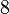$~8$, so the ratio of radiation pressure to gas pressure is,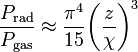$\frac{P_\mathrm{rad}}{P_\mathrm{gas}} \approx \frac{\pi^4}{15} \biggl( \frac{z}{\chi} \biggr)^3$ .

This means that radiation pressure will dominate over ideal gas pressure in any regime where,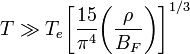$T \gg T_e \biggl[\frac{15}{\pi^4} \biggl(\frac{\rho}{B_F} \biggr) \biggr]^{1/3}$ ,

that is, whenever,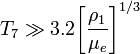$T_7 \gg 3.2 \biggl[\frac{\rho_1}{\mu_e} \biggr]^{1/3}$ ,

where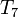$~T_7$ is the temperature expressed in units of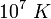$~10^7~K$ and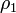$~\rho_1$ is the matter density expressed in units of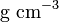$~\mathrm{g~cm}^{-3}$.

Second, note that the function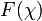$~F(\chi)$ can be written in a simpler form when examining regions of either very low or very high matter densities. Specifically — see our separate discussion of the Zero-Temperature Fermi gas — in the limit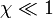$~\chi \ll 1$,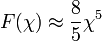$F(\chi) \approx \frac{8}{5} \chi^5$ ;

and in the limit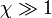$\chi \gg 1$,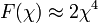$F(\chi) \approx 2 \chi^4$ .

Hence, at low densities (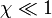$\chi \ll 1$),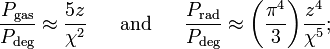$\frac{P_\mathrm{gas}}{P_\mathrm{deg}} \approx \frac{5 z}{ \chi^{2}} ~~~~~ \mathrm{and} ~~~~~ \frac{P_\mathrm{rad}}{P_\mathrm{deg}} \approx \biggl(\frac{\pi^4}{3}\biggr) \frac{z^4}{ \chi^5} ;$

and at high densities ($\chi \gg 1$),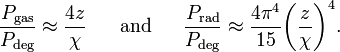$\frac{P_\mathrm{gas}}{P_\mathrm{deg}} \approx \frac{4z}{\chi} ~~~~~ \mathrm{and} ~~~~~ \frac{P_\mathrm{rad}}{P_\mathrm{deg}} \approx \frac{4 \pi^4}{15} \biggl( \frac{z}{\chi} \biggr)^4 .$

 © 2014 - 2019 by Joel E. Tohline |   H_Book Home   |   YouTube   | Context: | PGE | SR | Appendices: | Equations | Variables | References | Binary Polytropes | Ramblings | Images | Images (2016 Layout) | ADS |